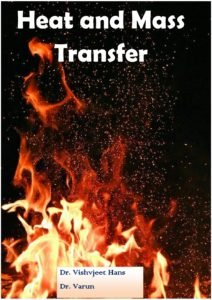You are here
Home > Book > ICAR eCourse > Agricultural Engineering > Heat and Mass Transfer (Agricultural Engg) PDF Book

# Heat and Mass Transfer (Agricultural Engg) PDF Book

Heat and Mass Transfer is a peer-reviewed scientific journal published by Springer. It serves the circulation of new developments in the field of basic research of heat and mass transfer phenomena, as well as related material properties and their measurements. Thereby applications to engineering problems are promoted.

Book Detail: Heat and Mass TransferLanguage: English

Pages: 267

Author: Dr. Vishvjeet Hans, Dr. Varun

Price: Free

All Agricultural Engineering PDF BookHeat and Mass Transfer

Course description: Introductory concepts, modes of heat transfer; Conduction- thermal conductivity of materials, general differential equation of conduction, one dimensional steady state conduction through plane and composite walls, tubes and spheres with and without heat generation, electrical analogy, Insulation materials, critical thickness of insulation; Fins- effectiveness, efficiency etc; Free and Forced Convection- Newton’s law of cooling, heat transfer coefficient in convection, dimensional analysis of free and forced convection, useful non dimensional numbers and empirical relationships for free and forced convection, equation of laminar boundary layer on flat plate and in a tube, laminar forced convection on a flat plate and in a tube, combined free and forced convection; Radiation- introduction, absorptivity, reflectivity and transmissivity, black body and monochromatic radiation, Planck’s law, Stefan-Boltzman law, Kirchoff’s law, grey bodies and emissive power, solid angle, intensity of radiation, radiation exchange between black surfaces, geometric configuration factor, heat transfer analysis involving conduction, convection and radiation by networks; Heat Exchangers- types, fouling factor, log mean temperature difference, heat exchanger performance, transfer units, heat exchanger analysis restricted to parallel and counter flow heat exchangers; Diffusion-steady state molecular diffusion in fluids at rest and in laminar flow, Fick’s law, mass transfer coefficients. Reynold’s analogy.

Subject: Heat and Mass Transfer (2+0) (32 Lessons) Content Developer: Vishavjeet Singh Hans

Module-I           Basic Concepts, Conductive Heat Transfer and Extended Surfaces

Lesson-1     Heat Transfer, Importance of Heat Transfer, modes of Heat Transfer: conduction, convection, radiation and Numerical Problems

Lesson-2     Conduction- thermal conductivity of materials, General heat conduction equation: Cartesian and cylindrical coordinates

Lesson-3     One dimensional steady state conduction through plane and composite walls, tubes and spheres without heat generation,

Lesson-4       One dimensional steady state conduction through plane and composite walls, tubes and spheres with heat generation

Lesson-5     Electrical analogy and Numerical Problems related to conduction

Lesson-6     Numericals on conduction

Lesson-7      Numericals on conduction

Lesson-8     Insulation   materials,   critical   thickness   of   insulation   and   Numerical Problems

Lesson-9     Types of Fins, Fin Applications, Heat Transfer through Fin of uniform cross-section,

Lesson-10   Special cases: Fin insulated at the end, fin sufficiently long Variation of Heat Loss from Fins with Length,

Lesson-11   Fin Efficiency and effectiveness, Problems on fins

### Module-II Convection

Lesson-12   Free and Forced Convection- Newton’s law of cooling, heat transfer coefficient in convection, Useful non dimensional numbers

Lesson-13   Dimensional analysis of free and forced convection

Lesson-14   Empirical relationships for free and forced convection

Lesson-15   Equation of laminar boundary layer on flat plate and in a tube

Lesson-16   Laminar forced convection on a flat plate

Lesson-17   Laminar forced convection in a tube, combined free and forced convection and Numerical problems

### Module-III Radiation

Lesson-18 Radiation, Basic Theory of Radiation Heat Transfer, Wave Theory,  Quantum Theory, Spectrum of Electromagnetic Radiation, Reflection, Absorption and Transmission of Radiation,

Lesson-19 Emission of Radiation, Black Body and Monochromatic Radiation, Monochromatic emissive power (Eλ) and Numerical Problems

Lesson-20 Planck’s law, Stefan-Boltzman law, Grey Body and Emissive Power of  Grey Body, Kirchoff’s Law of Radiation, Wien’s Displacement Law

Lesson-21 Solid Angle and Intensity of Radiation, Radiation Heat Transfer between Two Black Bodies

Lesson 22 Shape factor and its properties, Shape Factor of a Cavity with itself, Shape Factors of complex configurations derivable from perpendicular rectangles with common edge

Lesson-23 Radiation between two infinite parallel plates and proof of Kirchhoff’s law of Radiation, Radiation Shield

Lesson-24 Problems on Radiation

Food Engineering ICAR ECourse PDF Book

### Module-IV Heat Exchangers

Lesson-25 Introduction, Classification of Heat Exchangers, Logarithmic mean temperature difference

Lesson-26 Logarithmic mean temperature difference for parallel flow heat exchanger, counter and cross flow heat exchangers, Overall Heat Transfer Coefficient for a Plane wall and Double Pipe Heat Exchanger

Lesson-27 Logarithmic mean temperature difference for counter and cross flow heat exchangers, Fouling or scaling of heat exchanger and Numerical problems

Lesson-28 Heat exchanger performance in terms of Capacity ratio, Effectiveness and Number of transfer units, Effectiveness for parallel flow heat exchanger

Lesson-29   Effectiveness for counter flow heat exchanger and Numerical Problems Lesson-30   Numerical Problems related to heat exchanger performance

### Module-V Mass Transfer

Lesson-31   Introduction, Fick’s law of Diffusion, Mass Transfer Coefficients,

Lesson-32    Reynolds Analogy and Numerical problems

Feedback: After Reading these ICAR eCourse, please give your feedback for improve of the e-Course contents on this website. Click Here

Disclaimer: The information on this website does not warrant or assume any legal liability or responsibility for the accuracy, completeness or usefulness of the courseware contents.

The contents are provided free for noncommercial purpose such as teaching, training, research, extension and self learning.

If you are facing any Problem than fill form Contact Us

If you want share any article related Agriculture with us than send at info@agrimoon.com with your contact detail.

This site uses Akismet to reduce spam. Learn how your comment data is processed.